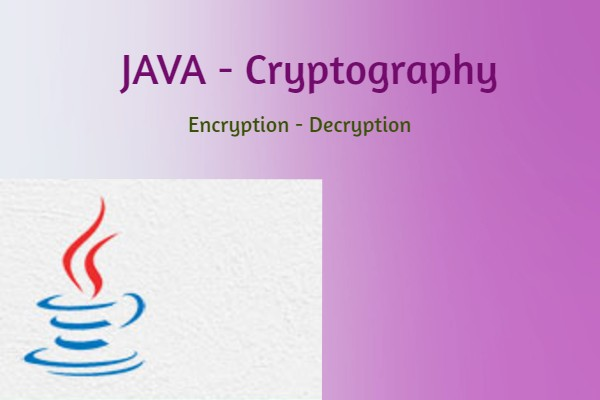To Learn about Encryption vs Decryption first we need to know about Cryptography.

What is meant by Cryptography?
Cryptography is a technique to provide the secure communication between any two nodes(client-server).

Encryption and Decryption are two functionalities used in Cryptography.

Encryption is a process of encoding the message in the form of unrecognized text.
Decryption is a process of decoding the encrypted message in to plain text message.

Why we need to use Encryption/Decryption ?
To protect our confidential data while sending on online

To do the Encryption/Decryption of data we need to have Cryptography keys.

We have different type of keys

Symmetric Key:
Symmetic key encryption are algorithms which use the same cryptographic keys on both side(encryption/decryption)

Asymmetric Key:
Asymmetric encryption uses 2 keys (public and private) for encryption. Publick key available for all and private key resides only at receiver side.

Public key:
Public key cryptography is an encryption system which is based on two pairs of keys. Public keys are used to encrypt messages for a receiver

Private key:
It may be a part of private/public key pair. It can be used in asymmetric encryption.

Pre-Shared Key:
PSK, a scret key which is shared between send/receiver side before using it.

The Cypher class is used in Encryption algorithm.

Process of Encryption/Decryption of data.

Step 1:
Create instance of Cypher class

Cipher cipher=Cipher.getInstance("AES");

Here creating the Cipher class instance with "Advanced Encryption Standard" algorithm.

Some encryption algorithms will work on different modes
An Encryption mode specifies the details about the encryption process.

Few Encryption modes

• EBC-Electronic Codebook
• CBC-Cipher Block Chaining
• CFB-Cipher Feedback
• OFB-Output Feedback
• CTR-Counter

Creating the Cipher instance with encryption mode.

Step 2:
Initialize Cipher

Cipher initalization can be done by init() method
cipher.init(Cipher.ENCRYPTION,key);

here Cipher.ENCRYPTION tells it making the encryption data.
and key the Encyprtion key.

for decryption process
cipher.init(Cipher.DECRYPTION,key);

Step 3:
Do Encryption/Decryption Process

The encryption/decryption of the data will make by the belwo methods of Cipher class
cipher.update()
cipher.doFinal()

byte[] plainText  = "Check the Encryption with AES".getBytes("UTF-8");
byte[] cipherText = cipher.doFinal(plainText);

here the cipherText will contains the encrypted data.

if we want to decrypt the encrypted data we need to initialize cipher with DECRYPTION mode.
cipher.init(Cipher.DECRYPTION,key);
byte[] plainText = cipher.doFinal(cipherText);